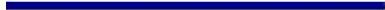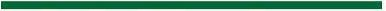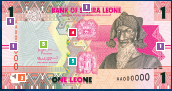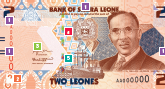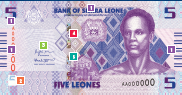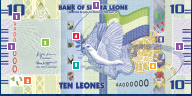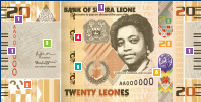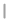Redenomination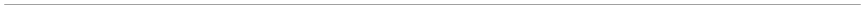Redenomination - Introduction
Also of Interest...
In simple terms, redenomination involves dividing the currency unit by a defined denominator and adapting that denominator to every amount in both notes and coins.
The first redenomination in Sierra Leone occurred in 1964 when the British West African Pound was converted into the current Leones and cents by dividing the West African Pound by 1.2 so that 6 pennies became 5 cents; 12 pennies (which constituted a shilling) became 10 cents; 120 pennies became 100 cents or 1 Leone, and 240 pennies became 2 Leones.
For this new redenomination exercise, requires dividing the currency by one thousand (1,000) resulting in the removal of three (3) zeros from the Leone.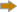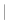Today :
Redenomination - Introduction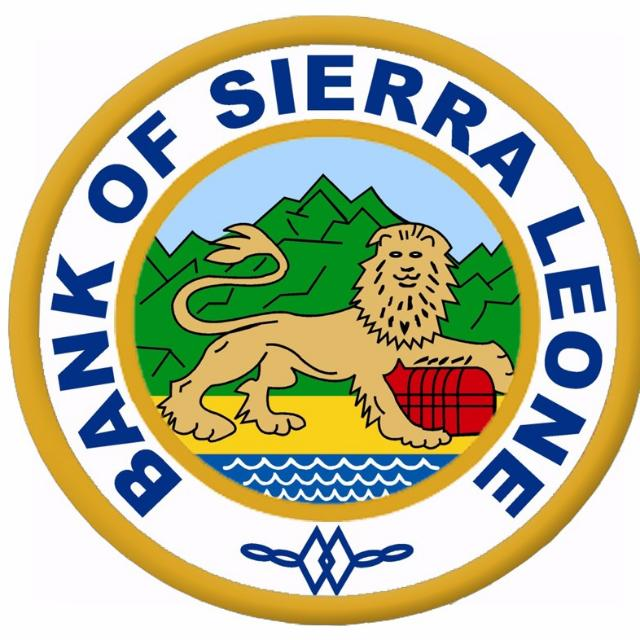BANK OF SIERRA LEONE